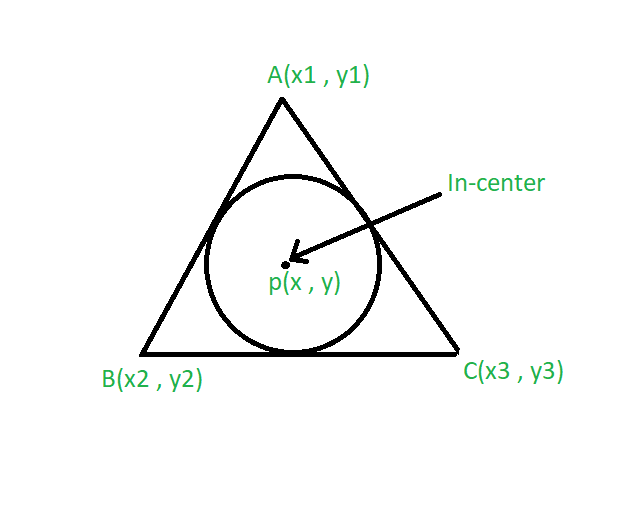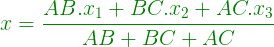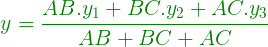Related Articles

# Program to Find the Incenter of a Triangle

• Last Updated : 03 May, 2021

Given the vertices of a triangle and length of its sides. A circle is inscribed in a triangle. The task is to find the incenter of a triangle.
Examples:

```Input: A(2, 2), B(1, 1), C(3, 1)
and AB = 2, BC = 1, AC = 1
Output: (2, 1.5)

Input: A(3, 3), B(1, 2), C(2, 2)
and AB = 3, BC = 2, AC = 2
Output: (2.5, 2.83)```Approach:

• The centre of the circle that touches the sides of a triangle is called its incenter.
• Suppose the vertices of the triangle are A(x1, y1), B(x2, y2) and C(x3, y3).
• Let the side AB = a, BC = b, AC = c then the coordinates of the in-center is given by the formula:••

Below is the implementation of the above approach:

## C++

 `// C++ program to find the``// incenter of a triangle``#include ``using` `namespace` `std;` `// Driver code``int` `main()``{``    ``// coordinate of the vertices``    ``float` `x1 = 2, x2 = 1, x3 = 3;``    ``float` `y1 = 2, y2 = 1, y3 = 1;``    ``float` `a = 2, b = 1, c = 1;` `    ``// Formula to calculate in-center``    ``float` `x = (a * x1 + b *``                   ``x2 + c * x3) / (a + b + c);``    ``float` `y = (a * y1 + b *``                   ``y2 + c * y3) / (a + b + c);` `    ``// System.out.print(setprecision(3));``    ``cout << ``"Incenter = "``         ``<< ``"("` `<< x << ``", "` `<< y << ``")"``;``    ``return` `0;``}` `// This code is contributed by 29AjayKumar`

## Java

 `// Java program to find the``// incenter of a triangle` `import` `java.util.*;``import` `java.lang.*;` `class` `GFG {` `    ``// Driver code``    ``public` `static` `void` `main(String args[])``    ``{``        ``// coordinate of the vertices``        ``float` `x1 = ``2``, x2 = ``1``, x3 = ``3``;``        ``float` `y1 = ``2``, y2 = ``1``, y3 = ``1``;``        ``float` `a = ``2``, b = ``1``, c = ``1``;` `        ``// Formula to calculate in-center``        ``float` `x``            ``= (a * x1 + b * x2 + c * x3) / (a + b + c);``        ``float` `y``            ``= (a * y1 + b * y2 + c * y3) / (a + b + c);` `        ``// System.out.print(setprecision(3));``        ``System.out.println(``"Incenter= "``                           ``+ ``"("` `+ x + ``", "` `+ y + ``")"``);``    ``}``}`

## Python3

 `# Python3 program to find the``# incenter of a triangle` `# Driver code` `# coordinate of the vertices``x1 ``=` `2``; x2 ``=` `1``; x3 ``=` `3``;``y1 ``=` `2``; y2 ``=` `1``; y3 ``=` `1``;``a ``=` `2``; b ``=` `1``; c ``=` `1``;` `# Formula to calculate in-center``x ``=` `(a ``*` `x1 ``+` `b ``*` `x2 ``+` `c ``*` `x3) ``/` `(a ``+` `b ``+` `c);``y ``=` `(a ``*` `y1 ``+` `b ``*` `y2 ``+` `c ``*` `y3) ``/` `(a ``+` `b ``+` `c);` `# System.out.print(setprecision(3));``print``(``"Incenter = ("``, x, ``","``, y, ``")"``);` `# This code is contributed``# by Akanksha Rai`

## C#

 `// C# program to find the``// incenter of a triangle` `using` `System;` `class` `GFG``{` `    ``// Driver code``    ``public` `static` `void` `Main()``    ``{``        ``// coordinate of the vertices``        ``float` `x1 = 2, x2 = 1, x3 = 3;``        ``float` `y1 = 2, y2 = 1, y3 = 1;``        ``float` `a = 2, b = 1, c = 1;` `        ``// Formula to calculate in-center``        ``float` `x``            ``= (a * x1 + b * x2 + c * x3) / (a + b + c);``        ``float` `y``            ``= (a * y1 + b * y2 + c * y3) / (a + b + c);` `        ``// System.out.print(setprecision(3));``        ``Console.WriteLine(``"Incenter= "``                        ``+ ``"("` `+ x + ``", "` `+ y + ``")"``);``    ``}``}` `// This code is contributed by vt_m.`

## Javascript

 ``
Output:
`Incenter= (2.0, 1.5)`

Attention reader! Don’t stop learning now. Get hold of all the important DSA concepts with the DSA Self Paced Course at a student-friendly price and become industry ready.  To complete your preparation from learning a language to DS Algo and many more,  please refer Complete Interview Preparation Course.

In case you wish to attend live classes with experts, please refer DSA Live Classes for Working Professionals and Competitive Programming Live for Students.

My Personal Notes arrow_drop_up